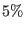# 1 Introduction

In the theory of general relativity, one can split the Riemann curvature tensor into the Ricci tensor defined by the Einstein equation and the Weyl curvature tensor.[1,2,3,4] Additionally, one can split the Weyl tensor into the electric part and the magnetic part, the so-called gravitoelectric/-magnetic fields, being due to some similarity to electrodynamical counterparts.[2,6,7,8,9] We describe the gravitoelectric field as the tidal (Newtonian) force,[9,10] but the gravitomagnetic field has no Newtonian analogy, called anti-Newtonian. Nonlocal characteristics arising from the Weyl curvature provides a description of the Newtonian force, although the Einstein equation describes a local dynamics of spacetime.[9,11] The Weyl curvature also includes an additional force: the gravitomagnetic field that is produced by the mass currents analogously an electric current generating a magnetic field. In fact, the theory of general relativity predicts two main concepts: gravitomagnetic fields and gravitational waves. Gravitation similar to electromagnetism propagates at identical speed, that provides a sounding analysis and a radiative description of force. We notice the Weyl tensor encoding the tidal force, a new force by its magnetic part, and a treatment of gravitational waves.

Determination of gravitational waves and gravitomagnetism (new force) is experimental tests of general relativity. Gravitational radiation of a binary system of compact objects has been proposed to be detected by a resonant bar or a laser interferometer in space,[14,15] such as the LIGO and VIRGO. A non-rotating compact object produces the standard Schwarzschild field, whereas a rotating body also generates the gravitomagnetic field. It has been suggested as a mechanism for the jet formation in quasars and galactic nuclei.[18,19] The resulting action of the gravitomagnetic fields and of the viscous forces implies that the formation of the accretion disk into the equatorial plane of the central body while the jets are ejected along angular momentum vector perpendicularly to the equatorial plane.[2,18] The gravitomagnetic field implies that a rotating body e.g. the Earth affects the motion of orbiting satellites. This effect has been recently measured using the LAGEOS I and LAGEOS II satellites. However, we may need counting some possible errors in the LAGEOS data. Using two recent orbiting geodesy satellites (CHAMP and GRACE), it has been reported confirmation of general relativity with a total error betweenand.[22,23,24]

In this paper, we describe kinematic and dynamic equations of the Weyl curvature variables, i.e., the gravitoelectric field as the relativistic generalization of the tidal forces and the gravitomagnetic field in a cosmological model containing the relativistic fluid description of matter. We use the convention based on. We denote the round brackets enclosing indices for symmetrization, and the square brackets for antisymmetrization. The organization of this paper is as follows. In Sec. 2, we introduce the 3 + 1 covariant formalism, kinematic quantities, and dynamic quantities in a hydrodynamic description of matter. In Sec. 3, we obtain constraint and propagation equations for the Weyl fields from the Bianchi and Ricci identities. In Sec. 4, rotation and distortion are characterized as wave solutions. In Sec. 5, we study a Newtonian model as purely gravitoelectric in an irrotational static spacetime and a perfect-fluid model, and an anti-Newtonian model as purely gravitomagnetic in a shearless static and perfect-fluid model. We see that both models are generally inconsistent with relativistic models, allowing no possibility for wave solutions. Section 6 provides a conclusion.

Ashkbiz Danehkar
2018-03-26